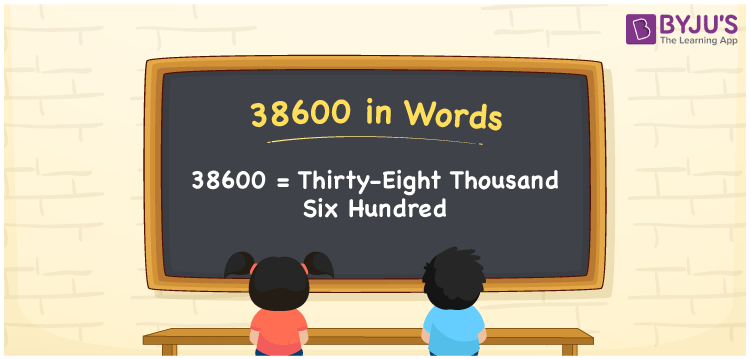# 38600 in Words

We can write 38600 in words as Thirty-eight thousand six hundred. If you bought an air cooler for Rs. 38600, you could say, “I bought an air cooler for Thirty-eight thousand six hundred rupees”. In this article, you will understand how to convert the cardinal number 38600 into words with the help of a place value chart and some interesting facts about 38600.

 38600 in words Thirty-eight thousand six hundred Thirty-eight thousand six hundred in Numbers 38600

## 38600 in English words

Generally, we express numbers in words using the English alphabet. Therefore, we can spell 38600 in English as “Thirty-eight thousand six hundred”.## How to Write 38600 in Words?

Writing a number in word form is simple when we use the place value chart. The below table represents the five-column place value chart as the number 38600 has five digits.

 Ten thousand Thousands Hundreds Tens Ones 3 8 6 0 0

Here, ones = 0, tens = 0, hundred = 6, thousands = 8, ten thousands = 2

Now, by expanding these digits, we get;

3 × Ten thousand + 8 × Thousand + 6 × Hundred + 0 × Ten + 0 × One

= 3 × 10000 + 8 × 1000 + 6 × 100 + 0 × 10 + 0 × 1

= 30000 + 8000 + 600

= Thirty thousand + Eight thousand + Six hundred

= Thirty-eight thousand six hundred

Therefore, 38600 in words = Thirty-eight thousand six hundred.

As we know, 38600 is a natural number that is the successor of 38599 and the predecessor of 38601.

38600 in words – Thirty-eight thousand six hundred

Is 38600 an odd number? – No

Is 38600 an even number? – Yes

Is 38600 a perfect square number? – No

Is 38600 a perfect cube number? – No

Is 38600 a prime number? – No

Is 38600 a composite number? – Yes

## Frequently Asked Questions on 38600 in Words

Q1

### How do you write an amount Rs. 38600 in words on a cheque?

We can write an amount of Rs. 38600 in words as “Thirty-eight thousand six hundred rupees”.
Q2

### What is 38600 in words?

Thirty-eight thousand six hundred represents the number 38600 in words.
Q3

### Find the value of 38600 – 26900.

38600 – 26900 = 11700 Therefore, the value of 38600 – 26900 is 11700, i.e., Eleven thousand seven hundred.